### Question 18. Balance the following equation stepwise. SO2(g) + H2S(aq) → S(s) + H2O (l)

Question 18.

Balance the following equation stepwise.

SO2(g) + H2S(aq) → S(s) + H2O (l)

Balanced equation: SO2(g) + 2H2S(aq)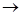3S(s) + 2H2O (l)

Explanation:

⇒ Step 1: Write the given unbalanced equation

SO2(g) + H2S(aq)S(s) + H2O (l)

⇒ Step 2: Compare the number of atoms of reactants with the number of atoms of products.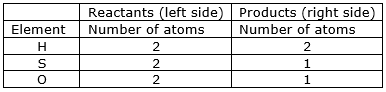⇒ Step 3: Now, let us consider oxygen atom. If we multiply 2 in the product (in H2O), we will get the equal number of atoms as in reactants (SO2)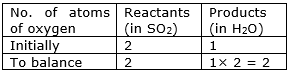⇒ Step 4: Write the resulting equation:

SO2 + H2S + 2H2O

⇒ Step 5: Now check whether the equation is balanced or not by comparing the atoms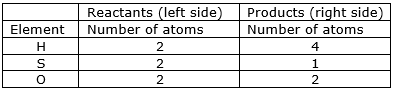We find that the equation is not balanced yet. As the number of

sulphur and hydrogen atoms are unequal on the two sides.

First balance the hydrogen number.

⇒ Step 6: Now, let us consider hydrogen atom. If we multiply 2 in the reactant (in H2S), we will get the equal number of atoms as in product (H2O)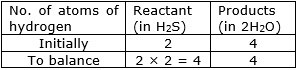⇒ Step 7: Write the resulting equation:

SO2 + 2H2S + 2H2O

⇒ Step 8: Now check whether the equation is balanced or not by comparing the atoms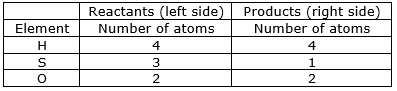We find that the equation is not balanced yet. As the number of

sulphur atom is unequal on the two sides.

⇒ Step 9: Now, let us consider sulphur atom. If we multiply 3 in the product (S), we will get the equal number of atoms as in reactants (SO2 and H2S)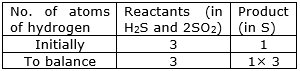⇒ Step 10: Write the resulting equation:

SO2 + 2H23S + 2H2O

⇒ Step 11: Now, compare the atoms of both the sides of all the elements. Write down the final balanced equation:

SO2 + 2H23S + 2H2O

## PDF FILE TO YOUR EMAIL IMMEDIATELY PURCHASE NOTES & PAPER SOLUTION. @ Rs. 50/- each (GST extra)

SUBJECTS

HINDI ENTIRE PAPER SOLUTION

MARATHI PAPER SOLUTION
SSC MATHS I PAPER SOLUTION
SSC MATHS II PAPER SOLUTION
SSC SCIENCE I PAPER SOLUTION
SSC SCIENCE II PAPER SOLUTION
SSC ENGLISH PAPER SOLUTION
SSC & HSC ENGLISH WRITING SKILL
HSC ACCOUNTS NOTES
HSC OCM NOTES
HSC ECONOMICS NOTES
HSC SECRETARIAL PRACTICE NOTES

2019 Board Paper Solution

HSC ENGLISH SET A 2019 21st February, 2019

HSC ENGLISH SET B 2019 21st February, 2019

HSC ENGLISH SET C 2019 21st February, 2019

HSC ENGLISH SET D 2019 21st February, 2019

SECRETARIAL PRACTICE (S.P) 2019 25th February, 2019

HSC XII PHYSICS 2019 25th February, 2019

CHEMISTRY XII HSC SOLUTION 27th, February, 2019

OCM PAPER SOLUTION 2019 27th, February, 2019

HSC MATHS PAPER SOLUTION COMMERCE, 2nd March, 2019

HSC MATHS PAPER SOLUTION SCIENCE 2nd, March, 2019

SSC ENGLISH STD 10 5TH MARCH, 2019.

HSC XII ACCOUNTS 2019 6th March, 2019

HSC XII BIOLOGY 2019 6TH March, 2019

HSC XII ECONOMICS 9Th March 2019

SSC Maths I March 2019 Solution 10th Standard11th, March, 2019

SSC MATHS II MARCH 2019 SOLUTION 10TH STD.13th March, 2019

SSC SCIENCE I MARCH 2019 SOLUTION 10TH STD. 15th March, 2019.

SSC SCIENCE II MARCH 2019 SOLUTION 10TH STD. 18th March, 2019.

SSC SOCIAL SCIENCE I MARCH 2019 SOLUTION20th March, 2019

SSC SOCIAL SCIENCE II MARCH 2019 SOLUTION, 22nd March, 2019

XII CBSE - BOARD - MARCH - 2019 ENGLISH - QP + SOLUTIONS, 2nd March, 2019

HSC Maharashtra Board Papers 2020

(Std 12th English Medium)

HSC ECONOMICS MARCH 2020

HSC OCM MARCH 2020

HSC ACCOUNTS MARCH 2020

HSC S.P. MARCH 2020

HSC ENGLISH MARCH 2020

HSC HINDI MARCH 2020

HSC MARATHI MARCH 2020

HSC MATHS MARCH 2020

SSC Maharashtra Board Papers 2020

(Std 10th English Medium)

English MARCH 2020

HindI MARCH 2020

Hindi (Composite) MARCH 2020

Marathi MARCH 2020

Mathematics (Paper 1) MARCH 2020

Mathematics (Paper 2) MARCH 2020

Sanskrit MARCH 2020

Important-formula

THANKS